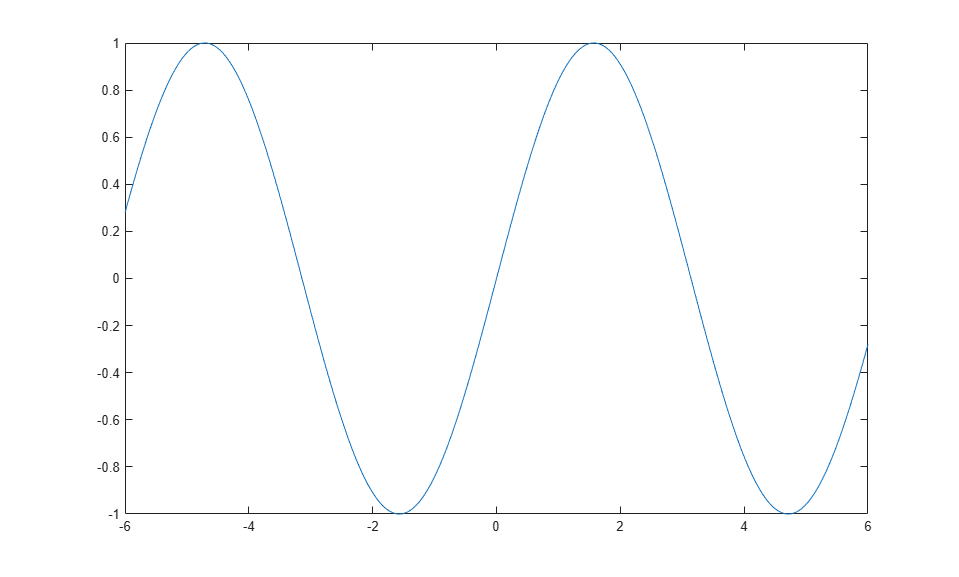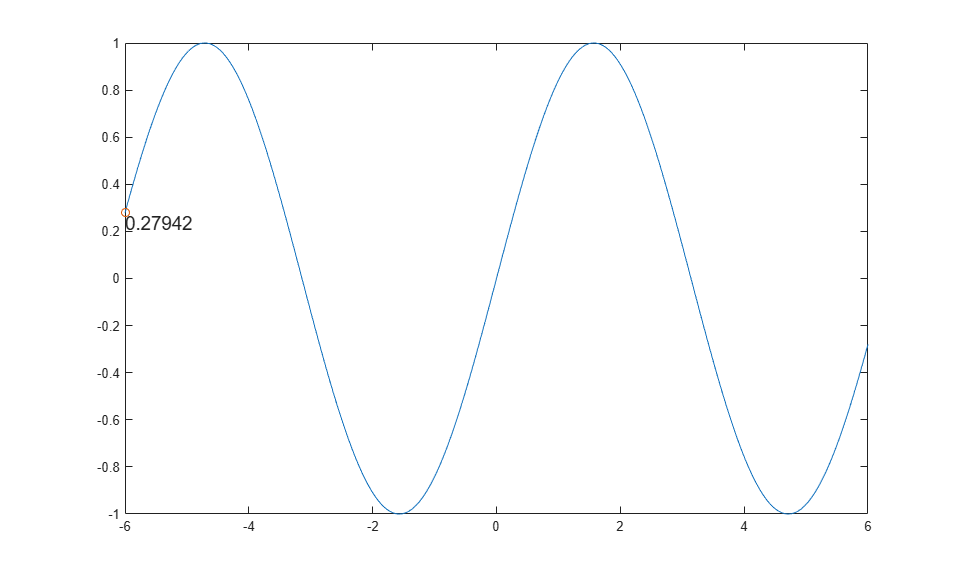# 沿着线条移动一组对象

```x = linspace(-6,6,1000); y = sin(x); plot(x,y) axis manual ``````ax = gca; h = hgtransform('Parent',ax); hold on plot(x(1),y(1),'o','Parent',h); hold off t = text(x(1),y(1),num2str(y(1)),'Parent',h,... 'VerticalAlignment','top','FontSize',14); ``````for k = 2:length(x) m = makehgtform('translate',x(k)-x(1),y(k)-y(1),0); h.Matrix = m; t.String = num2str(y(k)); drawnow end ```